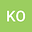A solution formula and the R-boundedness for the generalized Stokes resolvent problem in an infinite layer with Neumann boundary condition
•## Abstract

We consider the generalized Stokes resolvent problem in an infinite layer with Neumann boundary conditions. This problem arises from a free boundary problem describing the motion of incompressible viscous one-phase fluid flow without surface tension in an infinite layer bounded both from above and from below by free surfaces. We derive a new exact solution formula to the generalized Stokes resolvent problem and prove the $\mathscr{R}$-boundedness of the solution operator families with resolvent parameter $\lambda$ varying in a sector $\Sigma_{\varepsilon,\gamma_0}$ for any $\gamma_0>0$ and $0<\varepsilon<\pi/2$, where $\Sigma_{\varepsilon,\gamma_0} =\{ \lambda\in\mathbb{C}\setminus\{0\} \mid |\arg\lambda|\leq\pi-\varepsilon, \ |\lambda|>\gamma_0 \}$. As applications, we obtain the maximal $L_p$-$L_q$ regularity for the nonstationary Stokes problem and then establish the well-posedness locally in time of the nonlinear free boundary problem mentioned above in $L_p$-$L_q$ setting. We make full use of the solution formula to take $\gamma_0>0$ arbitrarily, while in general domains we only know the $\mathscr{R}$-boundedness for $\gamma_0\gg1$ from the result by Shibata. As compared with the case of Neumann-Dirichlet boundary condition studied by Saito, analysis is even harder on account of higher singularity of the symbols in the solution formula.

24 Jun 2020Submitted to Mathematical Methods in the Applied Sciences
27 Jun 2020Submission Checks Completed
27 Jun 2020Assigned to Editor
27 Jun 2020Reviewer(s) Assigned
10 Sep 2020Review(s) Completed, Editorial Evaluation Pending
10 Sep 2020Editorial Decision: Revise Major